# Supervised clustering of genes

## Abstract

### Background

We focus on microarray data where experiments monitor gene expression in different tissues and where each experiment is equipped with an additional response variable such as a cancer type. Although the number of measured genes is in the thousands, it is assumed that only a few marker components of gene subsets determine the type of a tissue. Here we present a new method for finding such groups of genes by directly incorporating the response variables into the grouping process, yielding a supervised clustering algorithm for genes.

### Results

An empirical study on eight publicly available microarray datasets shows that our algorithm identifies gene clusters with excellent predictive potential, often superior to classification with state-of-the-art methods based on single genes. Permutation tests and bootstrapping provide evidence that the output is reasonably stable and more than a noise artifact.

### Conclusions

In contrast to other methods such as hierarchical clustering, our algorithm identifies several gene clusters whose expression levels clearly distinguish the different tissue types. The identification of such gene clusters is potentially useful for medical diagnostics and may at the same time reveal insights into functional genomics.

## Background

Microarray technology allows the measurement of expression levels of thousands of genes simultaneously and is expected to contribute significantly to advances in fundamental questions of biology and medicine. We focus on the case where the experiments monitor the gene expression of different tissue samples, and where each experiment is equipped with an additional categorical outcome variable, describing, for example, a cancer type. An important problem in this setting is to study the relation between gene expression and tissue type. While microarrays monitor thousands of genes, it is assumed that only a few underlying marker components of gene subsets account for nearly all of the outcome variation - that is, determine the type of a tissue. The identification of these functional groups is crucial for tissue classification in medical diagnostics, as well as for understanding how the genome as a whole works.

As a first approach, unsupervised clustering techniques have been widely applied to find groups of co-regulated genes on microarray data. Hierarchical clustering [1,2] identifies sets of correlated genes with similar behavior across the experiments, but yields thousands of clusters in a tree-like structure. This makes the identification of functional groups very difficult. In contrast, self-organizing-maps  require a prespecified number and an initial spatial structure of clusters, but this may be hard to come up with in real problems. These drawbacks were improved by a novel graph theoretical clustering algorithm , but as with all other unsupervised techniques, it usually fails to reveal functional groups of genes that are of special interest in tissue classification. This is because genes are clustered by similarity only, without using any information about the experiment's response variables.

We focus here on supervised clustering, defined as grouping of variables (genes), controlled by information about the Y variables, that is, the tumor types of the tissues. Previous work in this field encompasses tree harvesting , a two-step method which consists first of generating numerous candidate groups by unsupervised hierarchical clustering. Then, the average expression profile of each cluster is considered as a potential input variable for a response model and the few gene groups that contain the most useful information for tissue discrimination are identified. Only this second step makes the clustering supervised, as the selection process relies on external information about the tissue types. An interesting supervised clustering approach that directly incorporates the response variables Y in the grouping process is the partial least squares (PLS) procedure [6,7], a tool often applied in the chemometrics literature, which in a supervised manner constructs weighted linear combinations of genes that have maximal covariance with the outcome. PLS has the drawback that the fitted components involve all (usually thousands of) genes, which makes them very difficult to interpret.

Here we present a promising new method for searching functional groups, each made up of only a few genes whose consensus expression profiles provide useful information for tissue discrimination. Like PLS, it is a one-step approach that directly incorporates the response variables Y into the grouping process, and is thus an algorithm for supervised clustering of genes. Because of the combinatorial complexity when clustering thousands of genes, we rely on a greedy strategy. It optimizes an empirical objective function that quickly and efficiently measures the cluster's ability for phenotype discrimination. Inspired by , we choose Wilcoxon's test statistic for two unpaired samples , refined by a novel second criterion, the margin function. Our supervised algorithm can be started with or without initial groups of genes, and then clusters genes in a stepwise forward and backward search, as long as their differential expression in terms of our objective function can be improved. This yields clusters typically made up of three to nine genes, whose coherent average expression levels allow perfect discrimination of tissue types. In an empirical study, the clusters show excellent out-of-sample predictive potential, and permutation and randomization techniques show that they are reasonably stable and clearly more than just a noise artifact. The output of our algorithm is thus potentially beneficial for cancer-type diagnosis. At the same time it is very accessible for interpretation, as the output consists of a very limited number of clusters, each summarizing the information about a few genes. Thus, it may also reveal insights into biological processes and give hints on explaining how the genome works.

We first describe our new algorithm for supervised clustering of gene-expression data and then apply the procedure to eight publicly available microarray datasets and test the results for their predictive potential, stability and relevance.

## Results and discussion

### Algorithm for supervised clustering of genes

This section presents an algorithm for supervised learning of similarities and interactions among predictor variables for classification in very high dimensional spaces, and hence is predestinated for searching functional groups of genes on microarray expression data.

#### The partitioning problem

Our basic stochastic model for microarray data equipped with categorical response is given by a random pair

(X, Y) with values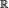p×where Xpdenotes a log-transformed gene-expression profile of a tissue sample, standardized to mean zero and unit variance.is the associated response variable, taking numeric values in= {0,1,..., K -1}. A usual interpretation is that Y codes for one of K cancer types. For simplicity, and a concise description of the algorithm, we first assume that K = 2, so that the response is binary. A generalization of the setting for multicategorical response (K > 2) is given below.

To account for the fact that not all p genes on the chip, but rather a few functional gene subsets, determine nearly all of the outcome variation and thus the type of a tissue, we model the conditional probability as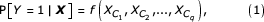where f(·) is a nonlinear function mapping fromqto [0,1], {C1,...,C q } with q << p are functional groups or clusters of genes which form a disjoint and usually incomplete partition of the index set: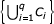{1,..., p} and C i C j = Ø, ij. Finally,denotes a 'representative' expression value of gene cluster C i . There are many possibilities to determine such group values, but as we would like to shape clusters that contain similar genes, a simple linear combination is an accurate choice (see [5,10]):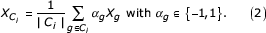Because of the use of log-transformed, mean-centered and standardized expression data, we, as a novel extension, allow the contribution of a particular gene g to the group valuealso to be given by its 'sign-flipped' expression value -X g . This means that we treat under- and overexpression symmetrically, and it prevents the differential expression of genes with different polarity (that is, one with low expression for class 0 and the other with low expression for class 1) from canceling out when they are averaged. But even by using such simple cluster expression values as in Equation 2, finding a partition of the index set {1,..., p} into subsets or clusters {C1,..., C q } that virtually determine the probability structure is still highly non-trivial and the design of a procedure that reveals the exact partition according to Equation 1 is too ambitious. Thus, we have developed a computationally intensive procedure that approximately solves Equation 1 and empirically yields good results.

#### Clustering with scores and margins

A practical heuristic for gene clustering is the cluster affinity search technique (CAST) . Our approach is algorithmically similar and also relies on growing the cluster incrementally by adding one gene after the other. Subsequent cleaning steps help us to remove spurious genes that were incorrectly added to the cluster at earlier stages. As in CAST, we repeat growth and removal until the cluster stabilizes, and then start a new cluster. The main, and very important, difference is that we do not augment (or shorten) the cluster by the gene that suits best (or least) into the current cluster in terms of an unsupervised similarity measure, but base our strategy for supervised clustering of genes on adding (or removing) the gene that improves the differential expression of the current cluster most, according to an empirical objective function for the representative group values from Equation 2. To be more explicit, we assume now that we are given n independent and identically distributed realizations

(x1, y1),.., (x n ,y n ), with x jpand y j {0,1},     (3)

of the random vector (X, Y), whose expression profiles x j are centered to mean zero and scaled to unit variance. The objective function needs to be a quantitative and efficiently computable measure of a cluster's ability to discriminate the tissues. As we aim for subsets of genes with accurate separation in binary problems, we rely on Wilcoxon's test statistic for two unpaired samples , which has been also applied as a nonparametric rank-based score function for genes in . The score of a single gene i is computed from its n-dimensional vector of observed values ξ i = (xi1,...,x in ),where x ij is the expression value of gene i for tissue j and N k represents the set of the n k tissues {1,...,n} being of type k {0,1}. The score uses information about the type of the tissues and is thus a criterion for supervised clustering. It can be interpreted as counting, for each experiment having response value 0, the number of tissues from class 1 that have smaller expression values, and summing up these quantities. Computing the score for a gene cluster C i , goes likewise via its observed representative values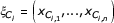. Viewing the score as Wilcoxon's test statistic, it allows the ordering of genes and clusters according to their potential significance for tissue discrimination. If the expression values of a particular gene or cluster yield exact separation of the classes, the expression values for all tissue samples having response 0 are uniformly lower than the ones belonging to class 1 or vice versa. In the former case, the score function returns its minimal value s min = 0, in the latter case the maximum score s max = n0n1 is assigned.

We rely on the use of log-transformed, mean-centered and standardized gene-expression data and thus need to prevent the averaging of two discriminatory genes with different polarity (that is, one with low expression for class 0 and the other with low expression for class 1) canceling out the differential expression of their mean. Therefore, we aim for low expression values pointing to class 0 for all genes, which is achieved by using the sign-flipped expression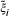for all genes i {1,...,p},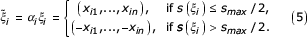The sign-flip is equivalent to setting α q = -1 in Equation 2 for all genes that tend to have lower expression values for the tissues of type 1 than for tissues of type 0. After the sign-flip, the scores of all individual genes i in the expression matrix are equal toand as all genes now have the same polarity, we can safely average them to compute group expression values. It is important to notice that the biological interpretation is not impeded by the sign-flips. Nevertheless, for interpretative purposes, the information about them should be recorded.

During the clustering process, we typically come across different gene or cluster expression vectors that have equal score (often zero) and hence the same quality according to our objective function. This is due to the discrete range of the score function. To achieve uniqueness in the decisions in which gene or cluster is optimal, we need a refinement of our objective function. We thus introduce the margin function, a continuous and real-valued measure for the strength of tissue discrimination of a sign-flipped gene-expression vector, where low expression values point towards the tissues of class 0,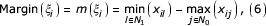where N0, N1 and x ij are as in Equation 4. The margin function is positive if, and only if, the score is zero andthen perfectly separates the tissues; otherwise it is negative. It measures the size of the gap between the lowest expression value from tissues with response 1, and the highest gene expression corresponding to class 0. The larger this gap, and hence the value of the margin function, the easier and clearer the discrimination of the two classes. The computation of the margin is again likewise for clusters via. Whenever various gene or cluster expression profiles have equal scores, their quality is judged by the margin function. Our objective function thus has two components. The score function is regarded as highest priority, whereas the margin function serves as the next highest priority criterion to achieve uniqueness.

#### The algorithm

Our clustering algorithm is detailed below.

1. 1.

Start with the entire p × n expression matrix X. Its rows are genes, and its columns are observations of two different tissue types, having zero mean and unit variance.

2. 2.

Determine the score of every gene i, that is, every n-dimensional row of observed expression values ξ i = (xi1,..., x in ) in X as in Equation 4. Flip the sign of each gene expression vector ξ i that has score s i ) >s max /2 by multiplying it with (-1),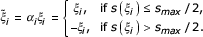This operation changes the score to s() = min(s i ), s max - s i )).

3. 3.

Composition of the starting values

4. (a)

If no initial cluster C is given, identify the gene i* having the lowest score s(). If more than one is found, the gene i* with the largest margin m() as in Equation 6 is chosen. Set the initial cluster mean ξ C equal to the expression vector (*) of the chosen gene.

5. (b)

If an initial cluster C is given, average the expression of the genes therein,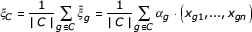1. 4.

Forward search

Average the current cluster expression profile ξ C with each individual gene i,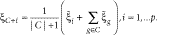Identify the winning gene i* as arg min i sC+i) that is, the gene that leads to the lowest score. If not unique, identify the winning gene i* as the one that optimizes score and margin; that is, i* = arg min i sC+i) as well as i* = arg max i mC+i).

2. 5.

Repeat step 4 until the identified gene i* is no longer accepted to enter the cluster. This is said to happen if the score of the updated cluster expression vector ξC+i* worsens, that is, sC+i*) >s C ), or if the score remains unchanged and the margin deteriorates, that is, sC+i*) = s C ) as well as mC+i*) <m C ).

3. 6.

Backward search

Exclude each gene i of the current cluster C separately, and average the expression vectors of the remaining genes,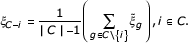Compute score and margin of each ξC-i. Identify (as in step 4) that gene i* whose exclusion optimizes the score, or if not unique, optimizes score and margin.

4. 7.

Repeat step 6 until the exclusion of the identified gene i* is (according to the formulation in step 5) no longer accepted.

5. 8.

Repeat steps 4-7 until the cluster converges and the objective function is optimal.

6. 9.

If more than one cluster C is desired, discard the genes in the former clusters from X and restart the algorithm at step 3 with the reduced, sign-flipped expression matrix.

The algorithm begins with the sign-flip operation described in Equation 5 to bring all genes to the same polarity. The clustering process can be started with or without initial gene clusters. If none are given, we start the procedure with the single gene that optimizes the objective function. Otherwise, the representative value of the starting cluster is determined. We then proceed by constructing the cluster incrementally. By searching among all genes, we merge and average the current cluster with one single gene, such that the augmented cluster optimizes our objective function, that is, has the lowest score or (in case of 'ties') the largest margin. The merging process is repeated until the objective function can no longer be improved. To remove spurious elements out of the current cluster, we then continue with a backward pruning stage, where genes are excluded step by step so that the objective function is optimized by every single removal. This cleaning stage aims to root out genes that were wrongly added to the cluster before. Accordingly, the forward and backward stages are repeated until the cluster converges, that is, when no further improvement of the objective function by adding or removing single genes is possible.

If one wishes to have more than q = 1 cluster for a binary class distinction, the genes forming the first cluster are discarded from the expression matrix, and the clustering process is restarted, again with or without an initial cluster. The algorithm's computations are feasible for dimensions p and sample sizes n which are clearly beyond today's common orders and hence also applicable for microarray experiments in the future. The computing time for searching q = 5 clusters in the binary leukemia dataset with n = 72 observations and p = 3,571 genes on a Linux PC with an Intel Pentium IV 1.6 GHz processor is about 5 seconds only. Software for the supervised clustering algorithm is available free as an R-Package at .

In summary, our cluster algorithm is a combination of variable (gene) selection for cluster membership and formation of a new predictor by possible sign-flipping and averaging the gene expressions within a cluster as in Equation 2. The cluster membership is determined with a forward and backward searching technique that optimizes the predictive score and margin criteria in Equations 4 and 6, which both involve the supervised response variables from the data.

#### Generalization for multiclass problems

Here we explain the extension of the supervised clustering algorithm to multicategory (K > 2) problems, where the response comprises more than two tissue types. We recommend comparing each response class separately against all other classes. This one-against-all approach for reduction to K binary problems is very popular in the machine-learning community, as many algorithms are solely designed for binary response. It works by definingand running K times the supervised clustering algorithm on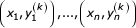as explained above. The interpretation is that we, as in Equation 1, model the conditional probability for discrimination of the kth class versus all the other response categories as depending on a few gene subsets only,where f k (·) are nonlinear functions mapping fromqto [0,1].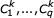are the q <<p functional groups of genes and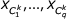are their representative group values, defined as in Equation 2. When the supervised clustering algorithm is applied to each of the K binary class distinctions, this results in totally K·q clusters, which can then be used to model the conditional probability for the K-class response,It is important to notice that instead of considering each class against all the other classes, many more ways to reduce a multi-class problem to multiple binary problems exist (see [12,13] for a thorough discussion). We assume that problem-dependent solutions that utilize deeper knowledge about the biological relation between the tissue types could be even more accurate for reducing multicategory problems to binary problems.

### Numerical results

#### Data

Leukemia dataset. This dataset contains gene expression levels of n = 72 patients either suffering from acute lymphoblastic leukemia (ALL, 47 cases) or acute myeloid leukemia (AML, 25 cases) and was obtained from Affymetrix oligonucleotide microarrays. For more information see ; the data are available at . Following exactly the protocol in , we preprocess the data by thresholding, filtering, a logarithmic transformation, and standardization, so that they finally comprise the expression values of p = 3,571 genes.

Breast cancer dataset. This dataset, described in , monitors p = 7,129 genes in 49 breast tumor samples. The data were obtained by applying the Affymetrix technology and are available at . We thresholded the raw data with a floor of 100 and a ceiling of 16,000 before applying a base 10 logarithmic transformation. Finally, each experiment was standardized to zero mean and unit variance. The response variable describes the status of the estrogen receptor (ER). According to , two samples failed to hybridize correctly and were excluded from their analysis. In five cases, two different clinical tests for determination of the ER status yielded conflicting results. These five plus another four randomly chosen samples were also separated from the rest of the data, so that a dataset of n = 38 samples remained, of which 18 were ER-positive and 20 ER-negative.

Colon cancer datase. In this dataset, expression levels of 40 tumor and 22 normal colon tissues for 6,500 human genes are measured using the Affymetrix technology. A selection of 2,000 genes with highest minimal intensity across the samples has been made in . The data are available at . As for all other datasets, we process these data further by carrying out a base 10 logarithmic transformation and standardizing each tissue sample to zero mean and unit variance across the genes.

Prostate cancer dataset. The raw data are available at  and comprise the expression of 52 prostate tumors and 50 non-tumor prostate samples, obtained using the Affymetrix technology. We use normalized and thresholded data as described in . We also excluded genes whose expression varied less than fivefold relatively, or less than 500 units absolutely, between the samples, leaving us with the expression of p = 6,033 genes. Finally, we applied a base 10 logarithmic transformation and standardized each experiment to zero mean and unit variance across the genes.

SRBCT dataset. This was described in  and contains gene-expression profiles for classifying small round blue-cell tumors of childhood (SRBCT) into four classes (neuroblastoma, rhabdomyosarcoma, non-Hodgkin lymphoma, Ewing family of tumors) and was obtained from cDNA microarrays. A training set comprising 63 SRBCT tissues, as well as a test set consisting of 20 SRBCT and 5 non-SRBCT samples are available at . Each tissue sample is associated with a thoroughly preprocessed expression profile of p = 2,308 genes, already standardized to zero mean and unit variance across genes.

Lymphoma dataset. This dataset is available at  and contains gene-expression levels of the K = 3 most prevalent adult lymphoid malignancies: 42 samples of diffuse large B-cell lymphoma (DLBCL, class 0), 9 observations of follicular lymphoma (FL, class 1), and 11 cases of chronic lymphocytic leukemia (CLL, class 2). The total sample size is n = 62, and the expression of p = 4,026 well-measured genes, preferentially expressed in lymphoid cells or with known immunological or oncological importance is documented. More information on these data can be found in . We imputed missing values and standardized the data as described in.

Brain tumor dataset. This dataset, presented in , contains n = 42 microarray gene expression profiles from K = 5 different tumors of the central nervous system, that is, 10 medulloblastomas, 10 malignant gliomas, 10 atypical teratoid/rhabdoid tumors (AT/RTs), 8 primitive neuro-ectodermal tumors (PNETs) and 4 human cerebella. The raw data were originated using the Affymetrix technology and are publicly available at . For data preprocessing, we followed the protocol in the supplementary information to . After thresholding, filtering, a logarithmic transformation and standardization of each experiment to zero mean and unit variance, a dataset comprising p = 5,597 genes remained.

National Cancer Institute (NCI) dataset. This comprises gene-expression levels of p = 5,244 genes for n = 61 human tumor cell lines which can be divided in K = 8 classes: seven breast, five CNS, seven colon, six leukemia, eight melanoma, nine non-small-cell lung carcinoma, six ovarian and nine renal tumors. A more detailed description of the data can be found at  and in . We work with preprocessed data as in .

#### Results from the supervised clustering algorithm

In this section we briefly describe the results obtained by applying the supervised clustering algorithm to the above datasets. Generally, the output of the clustering procedure is very promising. In all eight datasets we analyzed, comprising a total of 24 binary class distinctions, the average cluster expression x C always perfectly discriminates the two response classes (in multiclass problems, this is one class against the rest). Hence, the scores of all clusters are equal to zero. Moreover, the clusters have strongly positive margins, indicating that the different tissue types are clearly separated. As an example, Figure 1 shows impressively how well the average cluster expression vectors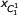and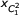discriminate between the three response classes of the lymphoma dataset. It is intuitively clear from Figure 1 that our cluster expression vectors x C are very suitable as predictor variables for the tissue types and they indeed allow for error-free classification on the training data and also yield good results on independent test datasets.

#### Permutation test

This section is concerned with assessing relevance and addresses the question of whether or not the promising output of the clustering procedure is a noise artifact. For this purpose, we explore quality measures of clusters generated from random-noise gene-expression data and compare them to the results obtained with the original data. As the distributions of the score function s(·) and the margin function m(·) on noise are not known, we rely on simulations. Let (y1,..., y n ) be the original set of responses. Then,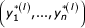is a 'shuffled' set of responses, constructed from the original response set by a random permutation for each l = 1,..., L. We then allocate an element of the permuted response to each of the (fixed) gene-expression profiles x i , giving us independent and identically distributed pairsas in Equation 3. The supervised clustering procedure is then applied L = 1,000 times on such data with randomly permuted responses. For every permuted set of responses, a single cluster (q = 1) was formed on the entire dataset and both its final score s*(l) and margin m*(l) were recorded (Tables 1, 2).

We explored the empirical distribution of the scores and margins from permuted data to judge whether the clusters found on the original datasets are of better quality than we would expect by chance. The results given in Figure 2 and in Tables 1 and 2 for a representative selection of data (see the legend to Table 1 for details of data selection) are very satisfactory. As outlined above, the scores s(0) on the original datasets altogether are equal to zero, with clearly positive margins m(0). The parameters on the randomly permuted data are worse: the final score s*(l) reached the minimal value of zero in 11% to 98% of the shuffling trials in different datasets (for example, 41% in Figure 2). These frequencies represent a non-significant result in our permutation test for the score function. However, this is not very troubling, as the final margins m*(l) for the permuted data were at best slightly positive, not indicating a clear separation of the randomly shuffled response classes. Values in the range of the margin in the original data were never achieved with any of the permuted data. This corresponds to a p-value of zero in the permutation test for our entire objective function consisting of score and margin. We thus can surely reject the hypothesis that the clusters found on the original data by our supervised algorithm are irrelevant and just a noise artifact. Moreover, we observed that the clusters from permuted data were much larger in size, clearly exceeding the typical size of between three to nine genes from non-permuted data. For example, permuted data gave a mean cluster size of 12.5 genes and a standard deviation (SD) of 3.2 for the AML/ALL distinction on the leukemia dataset.

The fact that the score has highly non-significant p-values is at first sight surprising. The reason for this is that the cluster expression values xC,jin Equation 2 are highly dependent among the samples j = 1,...,n via the responses y j in the supervisedly estimated cluster C = C(y1,...,y n ) and the sign coefficients α g = α g (y1,..., y n ). This strong interdependence causes the unusual phenomenon that the null-distribution, assuming no association between the expression values X and the response Y, has a substantial probability to score zero. The margin statistics in Equation 6 has much better power properties than the score.

#### Predictive potential

In this section, we will evaluate the predictive potential of the supervised clustering algorithm's output to see if it could successfully reveal functional groups of genes. A predictor or classifier for K different tissue types is a function C(·) that assigns a class label, based on an observed feature vector x. More precisely, the classification rule here will be based on average cluster expression values x= (xC10,..., xCqK-1) as K·q features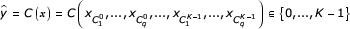In practice, the classifier is built from a learning set of tissues whose class labels are known. Subsequently it can be used to predict the class labels of new tissues with unknown outcome. There are various methods to build classification rules based on past experience and we restrict here on two relatively simple methods that are well suited for our purpose.

Nearest-neighbor classification. An easy to implement and, compared to more sophisticated methods, impressively competitive classifier for microarray data is the k-nearest-neighbor rule . It is based on a distance function d(·,·) for pairs xand x'of feature vectors. As we consider standardized gene-expression data here, the Euclidean distance function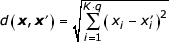is a reasonable choice. Then, for each new feature vector, the k closest feature vectors from the tissues in the learning data are identified and the predicted class is given by majority vote of the associated responses of these k closest neighbors. We found a choice of k = 1 neighbors to be appropriate, but more data-driven approaches via cross-validation for the determination of k would be possible.

Aggregated trees. Another approach that proved to be very fruitful in our setting is as follows: When knowing conditional probabilities p k (x) = P[Y(k) = 1|X= x], which specify how likely it is that a tissue with feature vector xbelongs to the kth or one of the other classes, the classifier function is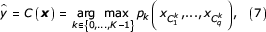meaning that a tissue is assigned to the class with highest probability. In practice, of course, we have to rely on estimated probabilities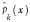. A method often applied to this task is the CART algorithm for fitting classification trees . The drawback when using it with our supervised clusters as input is that in case of perfect separation of the tissues in the training data, it only uses one (the first) component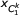of the feature vector xto determine conditional probabilities, and does not take into account any of the useful information about the remaining (q - 1) input variables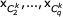. To improve the tree-based probability estimates, we design a novel technique based on plurality voting with classification trees, called aggregated trees. The idea is to fit q trees, one each with the q cluster expression profiles (components of the feature vector x) that have been found by our supervised algorithm for a particular binary class distinction. Each tree casts a weighted vote, i = 1,..., q, for response class k against the rest. Averaging then yields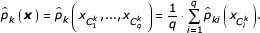as estimated conditional probabilities, which can be plugged into Equation 7 for maximum-likelihood classification.

Empirical study. Because, except for the leukemia and SRBCT data, no genuine test sets are available, our empirical study for exploring the classification potential is based on random divisions into learning and test set as well as leave-one-out cross-validation. For the latter, we set aside the ith tissue and carry out cluster identification and classifier fitting by considering only the remaining (n -1) data points. We then honestly predicti , the class label of the ith tissue sample and repeat this process for all data we have. Each observation is held out and predicted exactly once. We can determine the test-set error by calculating the fraction of predicted class labels which differ from the true class labels. Results for the nearest-neighbor and the aggregated tree classifier and varying number of clusters q are given in Table 3.

It is known from theory (see, for example ) that error rates from leave-one-out cross-validation have low bias but large variance. Estimating error rates by repeated random splitting of the data into training and (larger) test sets may be better in terms of mean squared error. In Table 4 we report misclassification rates which are based on N = 100 random divisions into a learning set comprising two thirds, and a test set containing the remaining third of all n data. We took care that the class proportions were roughly identical in learning and test set. Also, in every run here, both cluster identification and classifier construction are carried out on the training data, followed by honestly predicting the class labelsi for the test data with the two classifiers and various number of clusters q. The misclassification rate is then calculated as the averaged fraction of predicted class labels which differ from the true one.

We observe that the error estimates obtained from random splitting are on a slightly higher level than the ones from leave-one-out cross-validation. We also see that introducing some redundancy for the discrimination process by using additional clusters, that is, increasing q, yields better performance; but of course, a too large value of q would exhibit overfitting.

Comparison with classification using single genes. Does the use of averaged cluster expression profiles from our supervised algorithm improve the classification results compared to non-averaged, individual genes? To answer this important question, we also classified our datasets with exactly the same genes that were contained in the clusters, but did not average them. Instead of q average expression profiles, we then have roughly five times as many single genes as predictor variables. Misclassification rates from repeated random splitting are given in Table 5. We observe that the aggregated tree classifier yields in 54 of 56 cases better results with cluster averages than with individual genes as input. Also the nearest-neighbor classifier is in 43 out of 56 cases better when used in conjunction with clusters than with single genes. Note that since the events are not independent, we cannot use a binomial test for the null hypothesis of equal performance between clusters and single genes. An analysis of score and margin of the individual genes that were used in the clusters shows that most of them are not the strongest individually for predicting the tissue types, that is, they individually often only have mediocre scores and margins, but have very good predictive power as a group. So far, we gained evidence that our algorithm really identifies functional groups of genes whose average expression level has high explanatory power for the response classes.

Comparison with other studies. We now classify the breast cancer validation sample of , which contains four randomly chosen tissues plus five instances where two different clinical tests for determination of the ER status yielded conflicting results. We choose the nearest neighbor method with q = 3 clusters to be our classifier for the validation sample, as it had the best predictive potential on the n = 38 training data. Our predictions, shown in Table 6, always agree with the class label provided on the Proc Natl Acad Sci USA supporting information website , which corresponds to the outcome of the immunoblot assay method.

Not only the results on the validation sample are very convincing, but the cross-validation on the n = 38 training tissues is also error free. This is different from the results in  with precedent feature selection, singular value decomposition and Bayesian binary regression, where 7 of 9 tissues in the validation sample and 36 of 38 tissues in the training sample were accurately predicted. Moreover, our result confirms that the breast cancer expression matrix contains a strong signal for discriminating the ER status.

We next used our method to classify the original 34 test samples in the leukemia dataset. We applied the supervised clustering algorithm on the n = 38 training data, where we also fit the best predictor from our random splitting study (aggregated trees with q = 20 clusters as input features) as classifier for the independent sample. Our predictions turned out to be error-free, a result which can be directly compared to , where 29 of 34 observations were classified correctly by a weighted voting scheme. With support vector machines, results ranging between 30 to 32 correct classifications were reported . Moreover, a full leave-one-out cross-validation on the n = 38 training data (results not shown) resulted in perfect classification for various q values; also, the performance for cross-validation on the entire dataset with n = 72 observations is competitive, compared, for example, to .

The SRBCT data contains an additional test set of 20 SRBCT and 5 non-SRBCT samples. We first classified the 20 SRBCT tissues with the best classifier from the random splitting study on the n = 63 training samples, the nearest-neighbor method with q = 3 clusters as input. The predictions turned out to be error-free, approving the perfect classification with artificial neural networks and principal components as in , as well as the correct diagnosis obtained with multicategory support vector machines in . As aggregated trees and the one-nearest-neighbor classifier with q = 3 clusters as input are not well suited for assessing prediction strengths on the five non-SRBCT samples, we applied logistic discrimination and rejected every classification that was done with a probability lower than 0.95. All five non-SRBCT's did not exceed this threshold and were thus correctly rejected, whereas three of the twenty SRBCT tissues did not exceed it and could not confidently be classified either, though they were predicted correctly. Also, this result, as well as our error rate from leave-one-out cross-validation on the training data, which achieves the benchmark error rate of 0%, are consistent with [22,35]. This provides more evidence that our method can at least keep up with state-of-the-art classifiers such as neural networks or support vector machines.

The five remaining microarray studies do not contain genuine test sets and we thus compare our error rates from cross-validation and random splitting against the literature. The classification of tumor versus normal prostate tissue has been evaluated with leave-one-out cross-validation . After precedent feature selection, an accuracy of "greater than 90%" was obtained, a result that can be beaten by our error rate of 4.90%, which corresponds to five misclassifications in a total of 102 samples. The colon cancer dataset has already been considered by various authors, for example in , with classifiers based on single genes such as nearest neighbors and boosting in a cross-validation study. Our method does not clearly improve their results, although it seems to have an edge over them. However, we could not achieve a cross-validation error rate of 9.68%, as reported in  with support vector machines. The error rates on the lymphoma, brain tumor and NCI data provide evidence that our method, based on a one-against-all approach, does a good job in multiclass problems as well. On the lymphoma data we observe perfect classification, thus achieve the non-to-improve benchmark. On the brain tumor data, our minimal cross-validation error rate of 11.90% is superior to the 16.67% obtained in  with a weighted voting algorithm. Many more misclassifications occur on the NCI than on the other datasets, due to the large number of classes and their heterogeneity. However, when comparing our predictions to the results in a broad evaluation of classifiers on the NCI data , they prove to be very valuable. We consistently obtained mean error rates of less than 30% with random splitting, the optimum is 26.50% using aggregated trees with q = 20 clusters, whereas the best median error rates reported in  are in a range around 35% and higher (Table 7).

In summary, our predictions from simple classifiers based on the supervised clustering's output can easily keep up with sophisticated methods that are based on single genes, and as Table 7 shows, our supervised clusters beat the best reported results from the literature in four out of eight datasets. On three further datasets, we achieve the benchmark of perfect classification. The success of our method may be because the averaging of genes according to Equation 2 has a variance-reducing effect and yields more stable and accurate features for classification. As well as its good predictive potential, the cluster structure provided by our method is very accessible for biological interpretation and can be beneficial for functional genomics.

### Stability

The stability of the gene clusters detected by our supervised clustering algorithm is a critical issue. The output is much more useful for functional genomics if it remains unchanged for 'similar' input data. We use the bootstrap as a tool for assigning statistical significance, see . We assume n pairs of observations (x i ,y i ) with binary response y i {0,1}, from which we form a resampled gene expression dataset

(x1,y1)*,..., (x n ,y n )*

of length n by drawing with replacement from the original data pairs. We can then apply our supervised algorithm to extract clusters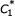,...,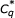out of these resampled data. For an empirical study, we generated L = 1,000 resampled gene-expression datasets of size n to explore the compositional variability of the first clusterin eight binary problems as detailed in the caption of Table 8.

We first analyze the variability in cluster size. The results, summarized in Table 8, show surprising stability across the eight different datasets. We observe that quite small clusters, typically made up of three to nine genes, were found. The SD in cluster size was fairly low in all eight datasets. As a next, and more difficult, step, we try to explore the compositional variability of the clusters. To give a rough overview which proportion of genes is actively present in the clustering process, we assess a confidence level to each individual gene i, which measures how likely it is to be clustered,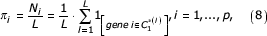where N i is the number of the L clusters that contain gene i. The numerical results given in Table 9 show that except for the colon tumor data, only a minority of genes ever entered a cluster. Also for the prostate and leukemia data this proportion was somewhat bigger, but still most of the genes never took part in the clustering process. More important, only a very small part of the genes is used frequently, that is more than 50 times in the 1,000 clusters. We conjecture that our supervised algorithm discriminates phenotypes with a small core of genes only, and in this sense it is reasonably stable.

We continue by assessing confidence levels to pairs of genes which gives a clue about pairwise interactions. We count the number N ij of clustersfound with our bootstrapped gene expression datasets that both contain the genes i and j, and then divide by the number of replicates L,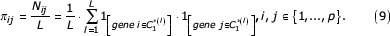These confidence levels not only give an idea how likely the pairs are, but also provide information for functional genomics, as we can now analyze whether pairs of genes preferentially enter clusters simultaneously or not. The number of hits N i for individual genes i follows a binomial(L i ) distribution (given the data), and for pairs (i,j) we have that N ij is binomial(L, π ij ) (we ignore here the fact that π i in Equation 8 and π ij in Equation 9 are computed with L = 1,000 replicates instead of the theoretical L = ∞. If there were no attraction or repulsion between genes, the joint probability π ij would be given by the product π i π j of the marginal probabilities. By calibrating the observed number of hits N ij with the binomial(L,N i N j /L) distribution under independence, we can test the hypothesis

H0 : π ij = π i π j ,

and compute the associated p-values. Low p-values indicate significant pairs of genes. Moreover, we also distinguish between two genes which are attracting (with N ij larger than expected under the null hypothesis), and which are repelling (with N ij lower than expected under H0). We implemented an empirical analysis based on L = 1,000 bootstrap trials, for pairs made up of the five genes with the highest confidence levels π i in the discrimination of lymphoma class 0 (DLBCL) from the other two phenotypes. Numerical results are summarized in Table 10, clone numbers and function of the genes are given in Table 11. Among the 10 pairs, several significant gene pairs that are strongly attracting or repelling are present; for example, genes 3786 and 3804 strongly attract each other. Moreover, 78% of the clusters that contained gene 3804 also included gene 3786, again signifying a special relation between these two. An interpretation of such facts in the framework of functional genomics is beyond the scope of this paper.

It is now tempting to extend this kind of analysis from pairs to tuples of third and higher orders. But estimating higher-order interactions will become very unreliable because of the limited amount of sample size n.

Our supervised clustering procedure can be understood as a generic method and allows alteration of various details according to the users' choice and specific demands. We also tried to improve the supervised clustering procedure ourselves with additional modifications, the most important of which are described here. The averaging of the gene expression in Equation 2 is specified by the arithmetic mean plus sign-flips, a very simple linear combination of genes, as it is impracticable to repeatedly optimize a general linear combination such as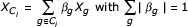during the clustering process. But theoretically, once the cluster algorithm has done its work, we could try to improve the discriminatory power of the actual cluster by numerically optimizing a weighted linear combination as above with respect to score and margin. In practice, we recognized that the numerical optimization was very difficult. If we started it with equal weights, they only changed slightly, and the objective function (this is, the margin) did not improve much. Because of this we favor the more simple method.

Since the margin function in Equation 6 is not scale-invariant, we also considered clustering with an adjusted margin. This means that we optimized the quotient of margin and within-group variation for a gene-expression vector ξ i = (xi1,...,x in ),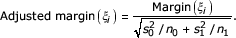Here, n k is the size andis the sample variance of class k {0,1}. While theoretically the size of the gap between the two response classes is meaningful only in relation to the within-group variance, the adjustment of the margin proved not to be very important in practice, owing to the use of standardized gene-expression data. It did not improve the predictive performance of the clusters and slightly decreased their stability. As it is common practice to standardize expression data, we recommend working with the non-adjusted margin.

Our algorithm, as described above, yields disjoint clusters of genes. To account for the fact that genes may function in multiple pathways, one could modify it as follows. First, run the clustering algorithm on the data, producing a first cluster; second, compute a probability estimate for P[Y = 1|X] for a two-class problem, for example, with probability-based classification methods or in a logistic model; third, reweight the data with weights as in the Real AdaBoost algorithm ; then return to the first step but now with reweighted data. Doing the loop q times produces q clusters, which are allowed to be non-disjoint.

We also explored the improvement of the supervised clustering algorithm by biasing it towards larger clusters. Specifically, we did not stop the forward search when score and/or margin first worsened, but continued as long as the objective function remained within a factor of the best. Our intention was that the objective function could improve again and reach even better values. As soon as the objective function once dropped below the tolerance (a factor times the best ever achieved value), we stopped the forward search and continued the algorithm with the cluster that yielded the best parameters ever. Although our first guess was that the biasing could result in larger clusters with clearer separation, it rarely ever had any effect in practice.

## Conclusions

We have proposed an algorithm for supervised clustering of genes from microarray experiments. Our procedure is potentially useful in the context of medical diagnostics, as it identifies groups of interacting genes that have high explanatory power for given tissue types, and which in turn can be used to accurately predict the class labels of new samples. At the same time, such gene clusters may reveal insights into biological processes and may be valuable for functional genomics.

In summary, our algorithm tries to cluster genes such that the discrimination of different tissue types is as simple as possible. It builds the clusters incrementally and relies on a fast, stepwise strategy that allows exhaustive searches among thousands of genes. More specifically, the aim is to identify sparse linear combinations of genes whose average expression level is uniformly low for one response class and uniformly high for the other class (es).

In empirical studies, the average cluster-expression profiles showed superior classification potential compared to other techniques where unclustered genes had been used. The clusters showed reasonable stability and there are several reasons that point towards their biological significance. They do not only contain the genes that are individually good, but groups of genes whose consensus expression profile is best with respect to the objective function. The predictive potential of the very same, unaveraged genes cannot keep up with the prediction potential of the corresponding cluster means. And, finally, an application of our algorithm to randomly permuted data shows that the identified structure is more than just a noise artifact.

An important task that remains to be addressed in future research is the generalization of the supervised clustering algorithm to quantitative response variables and to censored survival data. The fundamental idea of supervised clustering can be pursued again, but needs alternative objective functions that rank individual genes and gene clusters on the basis of their explanatory power for non-categorical response variables.

## References

1. 1.

Weinstein J, Myers T, O'Connor P, Friend H, Fornace A, Kohn K, Fojo T, Bates S, Rubinstein L, Anderson N, et al: An information-intensive approach to the molecular pharmacology of cancer. Science. 1997, 275: 343-349. 10.1126/science.275.5298.343.

2. 2.

Eisen M, Spellman P, Brown P, Botstein D: Cluster analysis and display of genome-wide expression patterns. Proc Natl Acad Sci USA. 1998, 95: 14863-14868. 10.1073/pnas.95.25.14863.

3. 3.

Tamayo P, Slonim D, Mesirov J, Zhu Q, Kitareewan S, Dmitrovsky E, Lander E, Golub T: Interpreting patterns of gene expression with self-organizing-maps: methods and application to hematopoietic differentiation. Proc Natl Acad Sci USA. 1999, 96: 2907-2912. 10.1073/pnas.96.6.2907.

4. 4.

Ben-Dor A, Shamir R, Yakhini Z: Clustering gene expression patterns. J Comput Biol. 1999, 6: 281-297. 10.1089/106652799318274.

5. 5.

Hastie T, Tibshirani R, Botstein D, Brown P: Supervised harvesting of expression trees. Genome Biol. 2001, 2: research0003.1-0003.12. 10.1186/gb-2001-2-1-research0003.

6. 6.

Nguyen D, Rocke D: Tumor classification by partial least squares using microarray gene expression data. Bioinformatics. 2002, 18: 39-50. 10.1093/bioinformatics/18.1.39.

7. 7.

Geladi P, Kowalski B: Partial least squares regression: a tutorial. Analyt Chim Acta. 1986, 185: 1-17. 10.1016/0003-2670(86)80028-9.

8. 8.

Park P, Pagano M, Bonetti M: A nonparametric scoring algorithm for identifying informative genes from microarray data. Pac Symp Biocomput. 2001, 52-63.

9. 9.

Wilcoxon F: Individual comparisons by ranking methods. Biometrics. 1945, 1: 80-83.

10. 10.

Hastie T, Tibshirani R, Eisen M, Alizadeh A, Levy R, Staudt L, Botstein D, Brown P: Gene shaving as a method of identifying distinct sets of genes with similar expression patterns. Genome Biol. 2000, 1: research0003.1-0003.21. 10.1186/gb-2000-1-2-research0003.

11. 11.

Marcel Dettling's website. [http://stat.ethz.ch/~dettling/supercluster.html]

12. 12.

Hastie T, Tibshirani R: Classification by pairwise coupling. Annls Statistics. 1998, 26: 451-471. 10.1214/aos/1028144844.

13. 13.

Allwein E, Schapire R, Singer Y: Reducing multiclass to binary: a unifying approach for margin classifiers. J Machine Learn Res. 2000, 1: 113-141. 10.1162/15324430152733133.

14. 14.

Golub T, Slonim D, Tamayo P, Huard C, Gassenbeek M, Coller H, Loh M, Downing J, Caliguri M, Bloomfield C, Lander E: Molecular classification of cancer: class discovery and class prediction by gene expression monitoring. Science. 1999, 286: 531-538. 10.1126/science.286.5439.531.

15. 15.

Whitehead Institute Center for Genomic Research: cancer genomics. [http://www-genome.wi.mit.edu/cancer]

16. 16.

Dudoit S, Fridlyand J, Speed T: Comparison of discrimination methods for the classification of tumors using gene expression data. J Am Stat Assoc. 2002, 97: 77-87. 10.1198/016214502753479248.

17. 17.

West M, Blanchette C, Dressman H, Huang E, Ishida S, Spang R, Zuzan H, Olson J, Marks J, Nevins J: Predicting the clinical status of human breast cancer by using gene expression profiles. Proc Natl Acad Sci USA. 2001, 98: 11462-11467. 10.1073/pnas.201162998.

18. 18.

Duke DNA Microarray Center. [http://mgm.duke.edu/genome/dna_micro/work/]

19. 19.

Alon U, Barkai N, Notterdam D, Gish K, Ybarra S, Mack D, Levine A: Broad patterns of gene expression revealed by clustering analysis of tumor and normal colon tissues probed by oligonucleotide arrays. Proc Natl Acad Sci USA. 1999, 96: 6745-6750. 10.1073/pnas.96.12.6745.

20. 20.

Colorectal Cancer Microarray Research. [http://microarray.princeton.edu/oncology/]

21. 21.

Singh D, Febbo P, Ross K, Jackson D, Manola J, Ladd C, Tamayo P, Renshaw A, D'Amico A, Richie J, et al: Gene expression correlates of clinical prostate cancer behavior. Cancer Cell. 2002, 1: 203-209. 10.1016/S1535-6108(02)00030-2.

22. 22.

Khan J, Wei J, Ringner M, Saal L, Ladanyi M, Westermann F, Berthold F, Schwab M, Antonescu C, Peterson C, Meltzer P: Classification and diagnostic prediction of cancer using gene expression profiling and artificial neural networks. Nat Med. 2001, 6: 673-679. 10.1038/89044.

23. 23.

National Human Genome Research Institute: microarray project. [http://www.nhgri.nih.gov/DIR/Microarray/Supplement]

24. 24.

Lymphoma/Leukemia Molecular Profiling Project Gateway. [http://llmpp.nih.gov/lymphoma/data/figure1]

25. 25.

Alizadeh A, Eisen M, Davis R, Ma C, Lossos I, Rosenwald A, Boldrick J, Sabet H, Tran T, Yu X, et al: Distinct types of diffuse large B-cell lymphoma identified by gene expression profiling. Nature. 2000, 403: 503-511. 10.1038/35000501.

26. 26.

Pomeroy S, Tamayo P, Gaasenbeek M, Sturla L, Angelo M, McLaugh-lin M, Kim J, Goumnerova L, Black P, Lau C, et al: Prediction of central nervous system embryonal tumor outcome based on gene expression. Nature. 2002, 415: 436-442. 10.1038/415436a.

27. 27.

Stanford NCI60 Cancer Microarray Project. [http://genome-www.stanford.edu/nci60/]

28. 28.

Ross D, Scherf U, Eisen M, Perou C, Rees C, Spellman P, Iyer V, Jeffrey S, Van de Rijn M, Waltham M, et al: Systematic variation in gene expression patterns in human cancer cell lines. Nat Genet. 1999, 24: 227-235.

29. 29.

Fix E, Hodges J: Discriminatory analysis - nonparametric discrimination: consistency properties. Report 1951, No. 4. US Air Force School of Aviation Medicine, Random Field, TX. In Machine Recognition of Patterns. Edited by: Agrawala A. 1977, New York: IEEE Press

30. 30.

Breiman L, Friedman J, Olshen R, Stone C: Classification and Regression Trees. Wadsworth: Belmont. 1984

31. 31.

Ripley B: Pattern Recognition and Neural Networks. Cambridge: Cambridge University Press. 1996

32. 32.

Data Collection for: West et al. (September 18, 2001) Proc Natl Acad Sci USA 10.1073/pnas.201162998. [http://www.pnas.org/cgi/content/full/201162998/DC1]

33. 33.

Furey T, Cristianini N, Duffy N, Bednarski D, Schummer M, Haussler D: Support vector machine classification and validation of cancer tissue samples using microarray expression data. Bioinformatics. 2000, 16: 906-914. 10.1093/bioinformatics/16.10.906.

34. 34.

Ben-Dor A, Bruhn L, Friedman N, Nachman I, Schummer M, Yakhini Z: Tissue classification with gene expression profiles. J Comput Biol. 2000, 7: 559-583. 10.1089/106652700750050943.

35. 35.

Lee Y, Lee C: Classification of multiple cancer types by multi-category support vector machines using gene expression data. Technical Report 1051. Madison, WI: University of Wisconsin, Department of Statistics. 2002

36. 36.

Efron B, Tibshirani R: The problem of regions. Annls Statistics. 1998, 26: 1687-1718. 10.1214/aos/1024691353.

37. 37.

Friedman J, Hastie T, Tibshirani R: Additive logistic regression: a statistical view of boosting. Annls Statistics. 2000, 28: 337-407. 10.1214/aos/1016218222.

## Acknowledgements

We thank Jane Fridlyand for providing the preprocessed NCI data. Software is available at .

## Author information

Authors

### Corresponding author

Correspondence to Marcel Dettling.

## Rights and permissions

Reprints and Permissions

Dettling, M., Bühlmann, P. Supervised clustering of genes. Genome Biol 3, research0069.1 (2002). https://0-doi-org.brum.beds.ac.uk/10.1186/gb-2002-3-12-research0069

• Revised:

• Accepted:

• Published:

### Keywords

• Partial Little Square
• Current Cluster
• Permute Data
• Multiclass Problem
• Random Splitting